Carbonite dropping fps while in cata dungeons - WoWInterface

12-06-10, 08:19 PM   #1
Nensec
A Deviate Faerie Dragon
Join Date: Nov 2010
Posts: 14
Carbonite dropping fps while in cata dungeons

For some reasen when i have carbonite enabled inside an instance my fps drops to 15 or less, but a weird thing is when i open a right click menu the fps drop dissapears.

I havent had the chance yet to run an instance with lua errors on so will edit when i get more info

edit:
here is the culprit: http://screenshot.xfire.com/s/107807751-4.jpg

Last edited by Nensec : 12-06-10 at 08:52 PM.

12-07-10, 09:15 AM   #2
Talandor
A Murloc Raider
Join Date: Oct 2010
Posts: 4
i am also having the same problem any help???

12-07-10, 10:56 PM   #3
Kazragore
A Deviate Faerie Dragon
Join Date: May 2007
Posts: 14
Same here. Carbonite produces up to 500 errors in cata dungeons.

12-08-10, 08:28 PM   #4
Kazragore
A Deviate Faerie Dragon
Join Date: May 2007
Posts: 14
push, the errors suck... Carbonite spam these errors in a neverending loop:

Code:
```1x Carbonite-4.031\Carbonite.lua:5539: attempt to index field '?' (a nil value)
Carbonite-4.031\Carbonite.lua:3536: in function `Upd'
Carbonite-4.031\Carbonite.lua:26033: in function <Carbonite\Carbonite.lua:25992>

Locals:
self = <table> {
PlX = 2194.58334961
LTF = NxMapTip {}
TrM = <table> {}
MIMZ = <table> {}
MiF = <table> {}
IMA = true
IcS = 1
CWUMI = 18195
Tar = <table> {}
MPY = -1104.4822547494
RMI = 18195
MLY = -1509.16665039
BAT = 0.4
ToB = <table> {}
TrD = false
GIM = <table> {}
MMGI = true
BGIN = 0
ScW = 0.88134943423136
Si1 = 1.1718750261934
SDW = 0.88134943423136
TNUI = 1
Win1 = <table> {}
DPS1 = 1
MMCD = 36
GIMIFN = <table> {}
InI = 18195
TeF = <unnamed> {}
MIPF = <table> {}
MDF = <table> {}
CMBG = false
BAF = 0.4
MPX = 2728.6410922974
MLX = 2194.58334961
Lev = 7
DZS = 1
CuO1 = false
CWUON = 1
UTD1 = 0
PIM = <table> {}
StT = -46
EfS = 0.63999998569489
Gui = <table> {}
MMS = <table> {}
MMSC = <table> {}
TETA = false
BAF1 = 1
CuO = <table> {}
WHC = <table> {}
BGGB = true
WoH = <table> {}
ArP = 1
CoF = <table> {}
DPS = 1
PSX = 2728.6410922974
MaI3 = 1
MDO = <table> {}
IcF = <table> {}
GIMITI = <table> {}
MaS = 0.11323590317912
LCT = 0
MMO1 = false
PlF = <unnamed> {}
BGIM = <table> {}
MPXD = 2685.9928644024
StS = 1
ShU = true
Con = 8
TrP = <table> {}
TFS2 = <table> {}
BaS = 1
PRZX = 54.267728328705
MISM = <table> {}
VSD = <table> {}
DAS = 0.1
KiS = false
Zon = 0
Tic = 290
MPYD = -1150.0782215713
PlS = 0
PlD = 360
BaA = 0.4
Tra1 = <table> {}
MMGUD = 1
Frm = <unnamed> {}
TSF = <unnamed> {}
PRZY = 93.899345397949
MaH = 235.54288966842
PlY = -1509.16665039
Men = <table> {}
TiF1 = <table> {}
WoA = 0
INIF = <table> {}
GOp = <table> {}
MaW = 243.4720775233
LTFS = <table> {}
Sca = 1.1346237498548
MISH = <table> {}
LOp = <table> {}
H = 141
PSCT = 57078.659
Scr2 = false
ISF1 = <table> {}
Dat = <table> {}
ScD = 1.1346237498548
MISW = <table> {}
MiB = 6
PaX = 0
TiH = 0
UTD = 0
ArS = 0.23
PSY = -1104.4822547494
W = 150
MMOF = <table> {}
NWU = false
DRS = 1
INS = 1
MoD = -63.198494028121
MaI = 18195
MoE = true
PLD = 360
BASO = <table> {}
}
ela = 0.078000001609325
Nx = <table> {
UnE = <function> @ Carbonite\Carbonite.lua:15521:
Com1 = <table> {}
SMT = <function> @ Carbonite\Carbonite.lua:10568:
CaF = <function> @ Carbonite\Carbonite.lua:21626:
Sli = <table> {}
ToB = <table> {}
CCW = <function> @ Carbonite\Carbonite.lua:25953:
OU__1 = <function> @ Carbonite\Carbonite.lua:24895:
GICH1 = <function> @ Carbonite\Carbonite.lua:19421:
VERSIONCD = 0.4
prt = <function> @ Carbonite\Carbonite.lua:4308:
Soc = <table> {}
prV = <function> @ Carbonite\Carbonite.lua:22219:
GPOI = <table> {}
Loc = "deDE"
NXMapKeyScaleRestore = <function> @ Carbonite\Carbonite.lua:15048:
VERSIONINFO = 0.24
DOE1 = <function> @ Carbonite\Carbonite.lua:21940:
SeQ = <function> @ Carbonite\Carbonite.lua:3031:
U_TFII = <function> @ Carbonite\Carbonite.lua:16124:
Lis = <table> {}
slC = <function> @ Carbonite\Carbonite.lua:9473:
AME = <function> @ Carbonite\Carbonite.lua:20676:
VERSIONTRAVEL = 0.4
NXWatchKeyUseItem = <function> @ Carbonite\Carbonite.lua:8791:
NXMapKeyTogNormalMax = <function> @ Carbonite\Carbonite.lua:18552:
Fon = <table> {}
VERSIONCAP = 0.7
TTSTCZXY = <function> @ Carbonite\Carbonite.lua:20941:
OpD = <table> {}
GeQ = <function> @ Carbonite\Carbonite.lua:8410:
Tim1 = <function> @ Carbonite\Carbonite.lua:8328:
VERSIONHUDOPTS = 0.03
CRC = <function> @ Carbonite\Carbonite.lua:19146:
GICH = <function> @ Carbonite\Carbonite.lua:10945:
BUILD = 271
Ski = <table> {}
U_TCR = <function> @ Carbonite\Carbonite.lua:10668:
VCA
---

1x Carbonite-4.031\Carbonite.lua:5539: attempt to index field '?' (a nil value)
Carbonite-4.031\Carbonite.lua:3536: in function `Upd'
Carbonite-4.031\Carbonite.lua:26033: in function <Carbonite\Carbonite.lua:25992>

Locals:
self = <table> {
PlX = 2194.58334961
LTF = NxMapTip {}
TrM = <table> {}
MIMZ = <table> {}
MiF = <table> {}
IMA = true
IcS = 1
CWUMI = 18195
Tar = <table> {}
MPY = -1104.4822547494
RMI = 18195
MLY = -1509.16665039
BAT = 0.4
ToB = <table> {}
TrD = false
GIM = <table> {}
MMGI = true
BGIN = 0
ScW = 0.88134943423136
Si1 = 1.1718750261934
SDW = 0.88134943423136
TNUI = 1
Win1 = <table> {}
DPS1 = 1
MMCD = 36
GIMIFN = <table> {}
InI = 18195
TeF = <unnamed> {}
MIPF = <table> {}
MDF = <table> {}
CMBG = false
BAF = 0.4
MPX = 2728.6410922974
MLX = 2194.58334961
Lev = 7
DZS = 1
CuO1 = false
CWUON = 1
UTD1 = 0
PIM = <table> {}
StT = -45
EfS = 0.63999998569489
Gui = <table> {}
MMS = <table> {}
MMSC = <table> {}
TETA = false
BAF1 = 1
CuO = <table> {}
WHC = <table> {}
BGGB = true
WoH = <table> {}
ArP = 1
CoF = <table> {}
DPS = 1
PSX = 2728.6410922974
MaI3 = 1
MDO = <table> {}
IcF = <table> {}
GIMITI = <table> {}
MaS = 0.11323590317912
LCT = 0
MMO1 = false
PlF = <unnamed> {}
BGIM = <table> {}
MPXD = 2686.9199997914
StS = 1
ShU = true
Con = 8
TrP = <table> {}
TFS2 = <table> {}
BaS = 1
PRZX = 54.267728328705
MISM = <table> {}
VSD = <table> {}
DAS = 0.1
KiS = false
Zon = 0
Tic = 291
MPYD = -1149.0870049013
PlS = 0
PlD = 360
BaA = 0.4
Tra1 = <table> {}
MMGUD = 1
Frm = <unnamed> {}
TSF = <unnamed> {}
PRZY = 93.899345397949
MaH = 235.54288966842
PlY = -1509.16665039
Men = <table> {}
TiF1 = <table> {}
WoA = 0
INIF = <table> {}
GOp = <table> {}
MaW = 243.4720775233
LTFS = <table> {}
Sca = 1.1346237498548
MISH = <table> {}
LOp = <table> {}
H = 141
PSCT = 57078.659
Scr2 = false
ISF1 = <table> {}
Dat = <table> {}
ScD = 1.1346237498548
MISW = <table> {}
MiB = 6
PaX = 0
TiH = 0
UTD = 0
ArS = 0.23
PSY = -1104.4822547494
W = 150
MMOF = <table> {}
NWU = false
DRS = 1
INS = 1
MoD = -63.198494028121
MaI = 18195
MoE = true
PLD = 360
BASO = <table> {}
}
ela = 0.09400000423193
Nx = <table> {
UnE = <function> @ Carbonite\Carbonite.lua:15521:
Com1 = <table> {}
SMT = <function> @ Carbonite\Carbonite.lua:10568:
CaF = <function> @ Carbonite\Carbonite.lua:21626:
Sli = <table> {}
ToB = <table> {}
CCW = <function> @ Carbonite\Carbonite.lua:25953:
OU__1 = <function> @ Carbonite\Carbonite.lua:24895:
GICH1 = <function> @ Carbonite\Carbonite.lua:19421:
VERSIONCD = 0.4
prt = <function> @ Carbonite\Carbonite.lua:4308:
Soc = <table> {}
prV = <function> @ Carbonite\Carbonite.lua:22219:
GPOI = <table> {}
Loc = "deDE"
NXMapKeyScaleRestore = <function> @ Carbonite\Carbonite.lua:15048:
VERSIONINFO = 0.24
DOE1 = <function> @ Carbonite\Carbonite.lua:21940:
SeQ = <function> @ Carbonite\Carbonite.lua:3031:
U_TFII = <function> @ Carbonite\Carbonite.lua:16124:
Lis = <table> {}
slC = <function> @ Carbonite\Carbonite.lua:9473:
AME = <function> @ Carbonite\Carbonite.lua:20676:
VERSIONTRAVEL = 0.4
NXWatchKeyUseItem = <function> @ Carbonite\Carbonite.lua:8791:
NXMapKeyTogNormalMax = <function> @ Carbonite\Carbonite.lua:18552:
Fon = <table> {}
VERSIONCAP = 0.7
TTSTCZXY = <function> @ Carbonite\Carbonite.lua:20941:
OpD = <table> {}
GeQ = <function> @ Carbonite\Carbonite.lua:8410:
Tim1 = <function> @ Carbonite\Carbonite.lua:8328:
VERSIONHUDOPTS = 0.03
CRC = <function> @ Carbonite\Carbonite.lua:19146:
GICH = <function> @ Carbonite\Carbonite.lua:10945:
BUILD = 271
Ski = <table> {}
U_TCR = <function> @ Carbonite\Carbonite.lua:10668:
VCA
---

1x Carbonite-4.031\Carbonite.lua:5539: attempt to index field '?' (a nil value)
Carbonite-4.031\Carbonite.lua:3536: in function `Upd'
Carbonite-4.031\Carbonite.lua:26033: in function <Carbonite\Carbonite.lua:25992>

Locals:
self = <table> {
PlX = 2194.58334961
LTF = NxMapTip {}
TrM = <table> {}
MIMZ = <table> {}
MiF = <table> {}
IMA = true
IcS = 1
CWUMI = 18195
Tar = <table> {}
MPY = -1104.4822547494
RMI = 18195
MLY = -1509.16665039
BAT = 0.4
ToB = <table> {}
TrD = false
GIM = <table> {}
MMGI = true
BGIN = 0
ScW = 0.88134943423136
Si1 = 1.1718750261934
SDW = 0.88134943423136
TNUI = 1
Win1 = <table> {}
DPS1 = 1
MMCD = 36
GIMIFN = <table> {}
InI = 18195
TeF = <unnamed> {}
MIPF = <table> {}
MDF = <table> {}
CMBG = false
BAF = 0.4
MPX = 2728.6410922974
MLX = 2194.58334961
Lev = 7
DZS = 1
CuO1 = false
CWUON = 1
UTD1 = 0
PIM = <table> {}
StT = -44
EfS = 0.63999998569489
Gui = <table> {}
MMS = <table> {}
MMSC = <table> {}
TETA = false
BAF1 = 1
CuO = <table> {}
WHC = <table> {}
BGGB = true
WoH = <table> {}
ArP = 1
CoF = <table> {}
DPS = 1
PSX = 2728.6410922974
MaI3 = 1
MDO = <table> {}
IcF = <table> {}
GIMITI = <table> {}
MaS = 0.11323590317912
LCT = 0
MMO1 = false
PlF = <unnamed> {}
BGIM = <table> {}
MPXD = 2687.8471351804
StS = 1
ShU = true
Con = 8
TrP = <table> {}
TFS2 = <table> {}
BaS = 1
PRZX = 54.267728328705
MISM = <table> {}
VSD = <table> {}
DAS = 0.1
KiS = false
Zon = 0
Tic = 292
MPYD = -1148.0957882312
PlS = 0
PlD = 360
BaA = 0.4
Tra1 = <table> {}
MMGUD = 1
Frm = <unnamed> {}
TSF = <unnamed> {}
PRZY = 93.899345397949
MaH = 235.54288966842
PlY = -1509.16665039
Men = <table> {}
TiF1 = <table> {}
WoA = 0
INIF = <table> {}
GOp = <table> {}
MaW = 243.4720775233
LTFS = <table> {}
Sca = 1.1346237498548
MISH = <table> {}
LOp = <table> {}
H = 141
PSCT = 57078.659
Scr2 = false
ISF1 = <table> {}
Dat = <table> {}
ScD = 1.1346237498548
MISW = <table> {}
MiB = 6
PaX = 0
TiH = 0
UTD = 0
ArS = 0.23
PSY = -1104.4822547494
W = 150
MMOF = <table> {}
NWU = false
DRS = 1
INS = 1
MoD = -63.198494028121
MaI = 18195
MoE = true
PLD = 360
BASO = <table> {}
}
ela = 0.062000002712011
Nx = <table> {
UnE = <function> @ Carbonite\Carbonite.lua:15521:
Com1 = <table> {}
SMT = <function> @ Carbonite\Carbonite.lua:10568:
CaF = <function> @ Carbonite\Carbonite.lua:21626:
Sli = <table> {}
ToB = <table> {}
CCW = <function> @ Carbonite\Carbonite.lua:25953:
OU__1 = <function> @ Carbonite\Carbonite.lua:24895:
GICH1 = <function> @ Carbonite\Carbonite.lua:19421:
VERSIONCD = 0.4
prt = <function> @ Carbonite\Carbonite.lua:4308:
Soc = <table> {}
prV = <function> @ Carbonite\Carbonite.lua:22219:
GPOI = <table> {}
Loc = "deDE"
NXMapKeyScaleRestore = <function> @ Carbonite\Carbonite.lua:15048:
VERSIONINFO = 0.24
DOE1 = <function> @ Carbonite\Carbonite.lua:21940:
SeQ = <function> @ Carbonite\Carbonite.lua:3031:
U_TFII = <function> @ Carbonite\Carbonite.lua:16124:
Lis = <table> {}
slC = <function> @ Carbonite\Carbonite.lua:9473:
AME = <function> @ Carbonite\Carbonite.lua:20676:
VERSIONTRAVEL = 0.4
NXWatchKeyUseItem = <function> @ Carbonite\Carbonite.lua:8791:
NXMapKeyTogNormalMax = <function> @ Carbonite\Carbonite.lua:18552:
Fon = <table> {}
VERSIONCAP = 0.7
TTSTCZXY = <function> @ Carbonite\Carbonite.lua:20941:
OpD = <table> {}
GeQ = <function> @ Carbonite\Carbonite.lua:8410:
Tim1 = <function> @ Carbonite\Carbonite.lua:8328:
VERSIONHUDOPTS = 0.03
CRC = <function> @ Carbonite\Carbonite.lua:19146:
GICH = <function> @ Carbonite\Carbonite.lua:10945:
BUILD = 271
Ski = <table> {}
U_TCR = <function> @ Carbonite\Carbonite.lua:10668:
VCA
---

1x Carbonite-4.031\Carbonite.lua:5539: attempt to index field '?' (a nil value)
Carbonite-4.031\Carbonite.lua:3536: in function `Upd'
Carbonite-4.031\Carbonite.lua:26033: in function <Carbonite\Carbonite.lua:25992>

Locals:
self = <table> {
PlX = 2194.58334961
LTF = NxMapTip {}
TrM = <table> {}
MIMZ = <table> {}
MiF = <table> {}
IMA = true
IcS = 1
CWUMI = 18195
Tar = <table> {}
MPY = -1104.4822547494
RMI = 18195
MLY = -1509.16665039
BAT = 0.4
ToB = <table> {}
TrD = false
GIM = <table> {}
MMGI = true
BGIN = 0
ScW = 0.88134943423136
Si1 = 1.1718750261934
SDW = 0.88134943423136
TNUI = 1
Win1 = <table> {}
DPS1 = 1
MMCD = 36
GIMIFN = <table> {}
InI = 18195
TeF = <unnamed> {}
MIPF = <table> {}
MDF = <table> {}
CMBG = false
BAF = 0.4
MPX = 2728.6410922974
MLX = 2194.58334961
Lev = 7
DZS = 1
CuO1 = false
CWUON = 1
UTD1 = 0
PIM = <table> {}
StT = -43
EfS = 0.63999998569489
Gui = <table> {}
MMS = <table> {}
MMSC = <table> {}
TETA = false
BAF1 = 1
CuO = <table> {}
WHC = <table> {}
BGGB = true
WoH = <table> {}
ArP = 1
CoF = <table> {}
DPS = 1
PSX = 2728.6410922974
MaI3 = 1
MDO = <table> {}
IcF = <table> {}
GIMITI = <table> {}
MaS = 0.11323590317912
LCT = 0
MMO1 = false
PlF = <unnamed> {}
BGIM = <table> {}
MPXD = 2688.7742705695
StS = 1
ShU = true
Con = 8
TrP = <table> {}
TFS2 = <table> {}
BaS = 1
PRZX = 54.267728328705
MISM = <table> {}
VSD = <table> {}
DAS = 0.1
KiS = false
Zon = 0
Tic = 293
MPYD = -1147.1045715612
PlS = 0
PlD = 360
BaA = 0.4
Tra1 = <table> {}
MMGUD = 1
Frm = <unnamed> {}
TSF = <unnamed> {}
PRZY = 93.899345397949
MaH = 235.54288966842
PlY = -1509.16665039
Men = <table> {}
TiF1 = <table> {}
WoA = 0
INIF = <table> {}
GOp = <table> {}
MaW = 243.4720775233
LTFS = <table> {}
Sca = 1.1346237498548
MISH = <table> {}
LOp = <table> {}
H = 141
PSCT = 57078.659
Scr2 = false
ISF1 = <table> {}
Dat = <table> {}
ScD = 1.1346237498548
MISW = <table> {}
MiB = 6
PaX = 0
TiH = 0
UTD = 0
ArS = 0.23
PSY = -1104.4822547494
W = 150
MMOF = <table> {}
NWU = false
DRS = 1
INS = 1
MoD = -63.198494028121
MaI = 18195
MoE = true
PLD = 360
BASO = <table> {}
}
ela = 0.063000001013279
Nx = <table> {
UnE = <function> @ Carbonite\Carbonite.lua:15521:
Com1 = <table> {}
SMT = <function> @ Carbonite\Carbonite.lua:10568:
CaF = <function> @ Carbonite\Carbonite.lua:21626:
Sli = <table> {}
ToB = <table> {}
CCW = <function> @ Carbonite\Carbonite.lua:25953:
OU__1 = <function> @ Carbonite\Carbonite.lua:24895:
GICH1 = <function> @ Carbonite\Carbonite.lua:19421:
VERSIONCD = 0.4
prt = <function> @ Carbonite\Carbonite.lua:4308:
Soc = <table> {}
prV = <function> @ Carbonite\Carbonite.lua:22219:
GPOI = <table> {}
Loc = "deDE"
NXMapKeyScaleRestore = <function> @ Carbonite\Carbonite.lua:15048:
VERSIONINFO = 0.24
DOE1 = <function> @ Carbonite\Carbonite.lua:21940:
SeQ = <function> @ Carbonite\Carbonite.lua:3031:
U_TFII = <function> @ Carbonite\Carbonite.lua:16124:
Lis = <table> {}
slC = <function> @ Carbonite\Carbonite.lua:9473:
AME = <function> @ Carbonite\Carbonite.lua:20676:
VERSIONTRAVEL = 0.4
NXWatchKeyUseItem = <function> @ Carbonite\Carbonite.lua:8791:
NXMapKeyTogNormalMax = <function> @ Carbonite\Carbonite.lua:18552:
Fon = <table> {}
VERSIONCAP = 0.7
TTSTCZXY = <function> @ Carbonite\Carbonite.lua:20941:
OpD = <table> {}
GeQ = <function> @ Carbonite\Carbonite.lua:8410:
Tim1 = <function> @ Carbonite\Carbonite.lua:8328:
VERSIONHUDOPTS = 0.03
CRC = <function> @ Carbonite\Carbonite.lua:19146:
GICH = <function> @ Carbonite\Carbonite.lua:10945:
BUILD = 271
Ski = <table> {}
U_TCR = <function> @ Carbonite\Carbonite.lua:10668:
VCA
---```
and much more...

Last edited by Kazragore : 12-08-10 at 08:37 PM.

12-08-10, 08:35 PM   #5
kingfetty
A Kobold Labourer
Join Date: Oct 2008
Posts: 1
Yeah, mine is so bad that WoW actually asks to disable it when I enter a dungeon because it's making so many errors

 12-09-10, 12:43 PM #6 carboniteaddon A Pyroguard Emberseer Join Date: Feb 2008 Posts: 2,245 Try the beta version here, which fixes map errors in 2 instances: http://www.wowinterface.com/forums/s...ad.php?t=37462 It is the same error repeating, which will happen when code fails that is called on a timer or frame update. WoW will ask to disable an addon in that case, since rapidly repeating errors will lower FPS. __________________ faatal
12-09-10, 07:30 PM   #7
Nensec
A Deviate Faerie Dragon
Join Date: Nov 2010
Posts: 14
Still getting frame drops, different error this time

http://screenshot.xfire.com/s/107920980-4.jpg

The frame drops stop completely when i turn my camera with my right mouse button but start again if i happen to hover over my map

12-09-10, 11:06 PM   #8
Kazragore
A Deviate Faerie Dragon
Join Date: May 2007
Posts: 14
 Originally Posted by carboniteaddon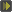Try the beta version here, which fixes map errors in 2 instances: http://www.wowinterface.com/forums/s...ad.php?t=37462 It is the same error repeating, which will happen when code fails that is called on a timer or frame update. WoW will ask to disable an addon in that case, since rapidly repeating errors will lower FPS.
Same issues with 4.032001 beta.

12-10-10, 05:41 AM   #9
Ratu
A Wyrmkin Dreamwalker
Join Date: May 2007
Posts: 58
 Originally Posted by carboniteaddonTry the beta version here, which fixes map errors in 2 instances: http://www.wowinterface.com/forums/s...ad.php?t=37462 It is the same error repeating, which will happen when code fails that is called on a timer or frame update. WoW will ask to disable an addon in that case, since rapidly repeating errors will lower FPS.
The FPS is still happening even with the new beta version. As i dont have any LUA errors to link, the spam of errors are still occurring with the newest beta version. Also, after logging out; disabling carbonite: and then back into any Cata dungeon, I get (no kidding) at least 80 FPS more with Carbonite disabled.

12-10-10, 06:41 AM   #10
XXXmahakalaXXX
A Murloc Raider
Join Date: Jan 2010
Posts: 6
 Originally Posted by NensecFor some reasen when i have carbonite enabled inside an instance my fps drops to 15 or less, but a weird thing is when i open a right click menu the fps drop dissapears. I havent had the chance yet to run an instance with lua errors on so will edit when i get more info edit: here is the culprit: http://screenshot.xfire.com/s/107807751-4.jpg
can confirm this

 12-10-10, 01:08 PM #11 carboniteaddon A Pyroguard Emberseer Join Date: Feb 2008 Posts: 2,245 I found the problem. It was a typo in a file. I will have a new beta out today with the fix. __________________ faatal
 12-10-10, 04:30 PM #12 carboniteaddon A Pyroguard Emberseer Join Date: Feb 2008 Posts: 2,245 Try 4.032002 beta: http://www.wowinterface.com/forums/s...ad.php?t=37462 __________________ faatal
12-11-10, 12:01 PM   #13
Nensec
A Deviate Faerie Dragon
Join Date: Nov 2010
Posts: 14
By the looks of things the problems have been fixed. i am running stonecore ( the place i had the most issues with ) now with carbonite on and there are no frame drops

12-30-10, 12:47 PM   #14
Nensec
A Deviate Faerie Dragon
Join Date: Nov 2010
Posts: 14
little update: this same problem now happens in raid instances

01-01-11, 08:08 AM   #15
Booga71
A Kobold Labourer
Join Date: Mar 2010
Posts: 1
 Originally Posted by Nenseclittle update: this same problem now happens in raid instances
I can confirm this, I am dropping to 15 fps when entering Blackrock when Carbonite is enabled.

01-01-11, 09:19 AM   #16
psychomuse
A Kobold Labourer
Join Date: Dec 2009
Posts: 1
screen freezes

I have noticed very short (1/2 second) screen freezes or severe reductions in fps whenever spells proc or I loot or accept quests. During these moments, the screen will seem as if it is stuck for a 1/2 second or so. I tried all types of video and audio adjustments (I thought maybe it was an audio issue since there were always event sounds that play during these things as well). Nothing worked. That is, until I started disabling addons one by one, only to find that the problem was completely resolved by disabling Carbonite alone. All of these issues only seem to occur while I am in areas that are part of the new Cata content. I am sure that Carbonite is the culprit. I hope this can be fixed because I don't know how I can play WoW without it!

01-01-11, 01:06 PM   #17
Jigain
A Molten Giant
Join Date: Jul 2009
Posts: 733
And did you try with Carbonite as the only enabled addon?
__________________01-04-11, 01:30 AM #18 carboniteaddon A Pyroguard Emberseer Join Date: Feb 2008 Posts: 2,245 After installing any version of Carbonite, you should check the version number in the addon list to ensure the files were copied successfully to the correct location. Press ESC, click Interface, click Help and make sure "Display LUA errors" is checked, then log out and back in. Report any errors that appear. Please state the version of Carbonite you are using in the bug report. You can leave this on permanently. It will not effect game play and alert you to addon problems. __________________ faatal
01-06-11, 02:32 PM   #19
Antelope
A Deviate Faerie Dragon
Join Date: Sep 2007
Posts: 13
 Originally Posted by carboniteaddonI found the problem. It was a typo in a file. I will have a new beta out today with the fix.
Any chance you could share with us what the typo was so we can search for it? I am getting thousands of LUA errors whenever I enter Blackwing Descent and am using the newest release of carbonite.

01-06-11, 06:59 PM   #20
Yelina
An Aku'mai Servant
Join Date: Feb 2007
Posts: 37
Isn't it much more simple to just delete all the carbonite folders from your addon folder.
And after that install the folders from the latest version of Carbonite.
Then you don't need to search for a typo in a file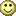WoWInterface » Carbonite dropping fps while in cata dungeons

 Thread Tools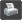Show Printable Version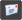Email this Page Display Modes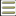Linear Mode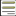Switch to Hybrid Mode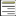Switch to Threaded Mode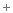Posting Rules You may not post new threads You may not post replies You may not post attachments You may not edit your posts vB code is On Smilies are On [IMG] code is On HTML code is Off